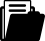Meine Position
i
Ein Tipp vom Eldar Team
Je mehr Sie bestellen, desto grösser Ihr Rabatt
LieferbarBuch
Buch
Fachbuch
2012

# Mathematical Foundations of Quantum Statistical Mechanics

## Continuous Systems

Autor/-in
ISBN
EAN
978-94-010-4083-9
9789401040839
Artikel-Nr.
ZR33597
Kostenloser Versand
Rabatt
-11.5
%
CHF 150.00
CHF
132.68
Anzahl
1
Maximale
Lieferzeit
20
Arbeitstage
Freitag
31.12.2021Beschreibung
This monograph is devoted to quantum statistical mechanics. It can be regarded as a continuation of the book "Mathematical Foundations of Classical Statistical Mechanics. Continuous Systems" (Gordon & Breach SP, 1989) written together with my colleagues V. I. Gerasimenko and P. V. Malyshev. Taken together, these books give a complete pre sentation of the statistical mechanics of continuous systems, both quantum and classical, from the common point of view. Both books have similar contents. They deal with the investigation of states of in finite systems, which are described by infinite sequences of statistical operators (reduced density matrices) or Green's functions in the quantum case and by infinite sequences of distribution functions in the classical case. The equations of state and their solutions are the main object of investigation in these books. For infinite systems, the solutions of the equations of state are constructed by using the thermodynamic limit procedure, accord ing to which we first find a solution for a system of finitely many particles and then let the number of particles and the volume of a region tend to infinity keeping the density of particles constant. However, the style of presentation in these books is quite different.Stichwörterdensitydifferential equationmechanicsstatistical mechanicssuperconductivity
Zielgruppe
Research
Inhaltsverzeichnis
Introduction. 1: Evolution of States of Quantum Systems of Finitely Many Particles. 1. Principal Concepts of Quantum Mechanics. 2. Evolution of States of Quantum Systems with Arbitrarily Many Particles. 3. Evolution of States in the Heisenberg Representation and in the Interaction Representation. Mathematical Supplement I. References. 2: Evolution of States of Infinite Quantum Systems. 4. Bogolyubov Equations for Statistical Operators. 5. Solution of the Bogolyubov Equations. 6. Gibbs Distributions. Mathematical Supplement II. Mathematical Supplement III. References. 3: Thermodynamic Limit. 7. Thermodynamic Limit for Statistical Operators. 8. Statistical Operators in the Case of Quantum Statistics. 9. Bogolyubov's Principle of Weakening of Correlations. Mathematical Supplement IV. References. 4: Mathematical Problems in the Theory of Superconductivity. 10. Fröhlich Model. 11. Bogolyubov's Compensation Principle for `Dangerous' Diagrams. Compensation Equations. 12. Bardeen--Cooper--Schrieffer (BCS) Hamiltonian. 13. Microscopic Theory of Superfluidity. Mathematical Supplement V. References. 6: Green's Functions. 14. Green's Functions. Equations for Green's Functions. 15. Investigation of the Equations for Green's Functions in the Theory of Superconductivity and Superfluidity. 16. Green's Functions in the Thermodynamic Limit. References. 6: Exactly Solvable Models. 17. Description of the Hamiltonians of Model Systems. 18. Functional Spaces of Translation--Invariant Function. 19. ModelHamiltonians in the Spaces of Translation Invariant Functions. 20. Model BCS Hamiltonian in the Space hT. Equivalence of General and Model Hamiltonians in the Space of Pairs. 21. Equations for Green's Functions and their Solutions. Mathematical Supplement VI. References. 7: Quasiaverages. Theorem on Singularities of Green's Functions of 1/q2-Type. 22. Quasiaverages. 23. Green's Functions and their Spectral Representations. 24. Theorem on Singularities of Green's Functions of 1/q2-Type. References. Subject Index.Herausgeber/-in
Publikation
Niederlande
14.10.2012Sprache
EnglischFormat
Softcover
445 Seiten
24 cm
(Höhe)
16 cm
(Breite)
735 g
(Gewicht)Weitere FormateVersand
Kostenloser Versand: Schweiz & Liechtenstein
Für den Versand nach Deutschland oder Frankreich werden die Versandgebühren der Schweizerischen Post berechnet. Diese werden Ihnen im Warenkorb für Ihre gesamte Bestellung berechnet.ZahlungsartenBestellen Sie einfach auf Rechnung oder bezahlen Sie bequem und gebührenfrei mit Twint oder Kreditkarte.Passende Themen
Zurück
Zum Start